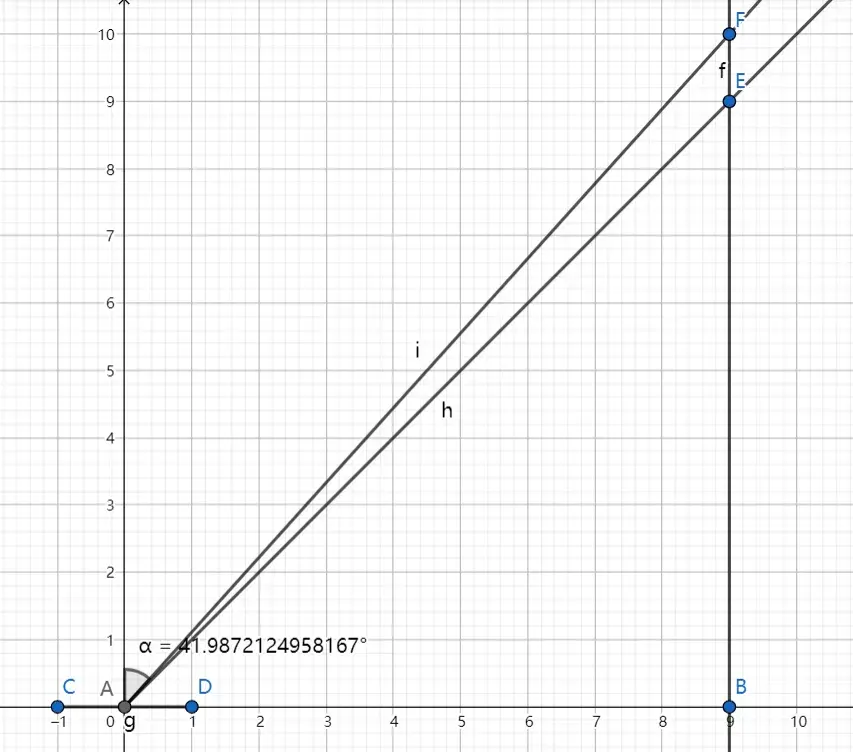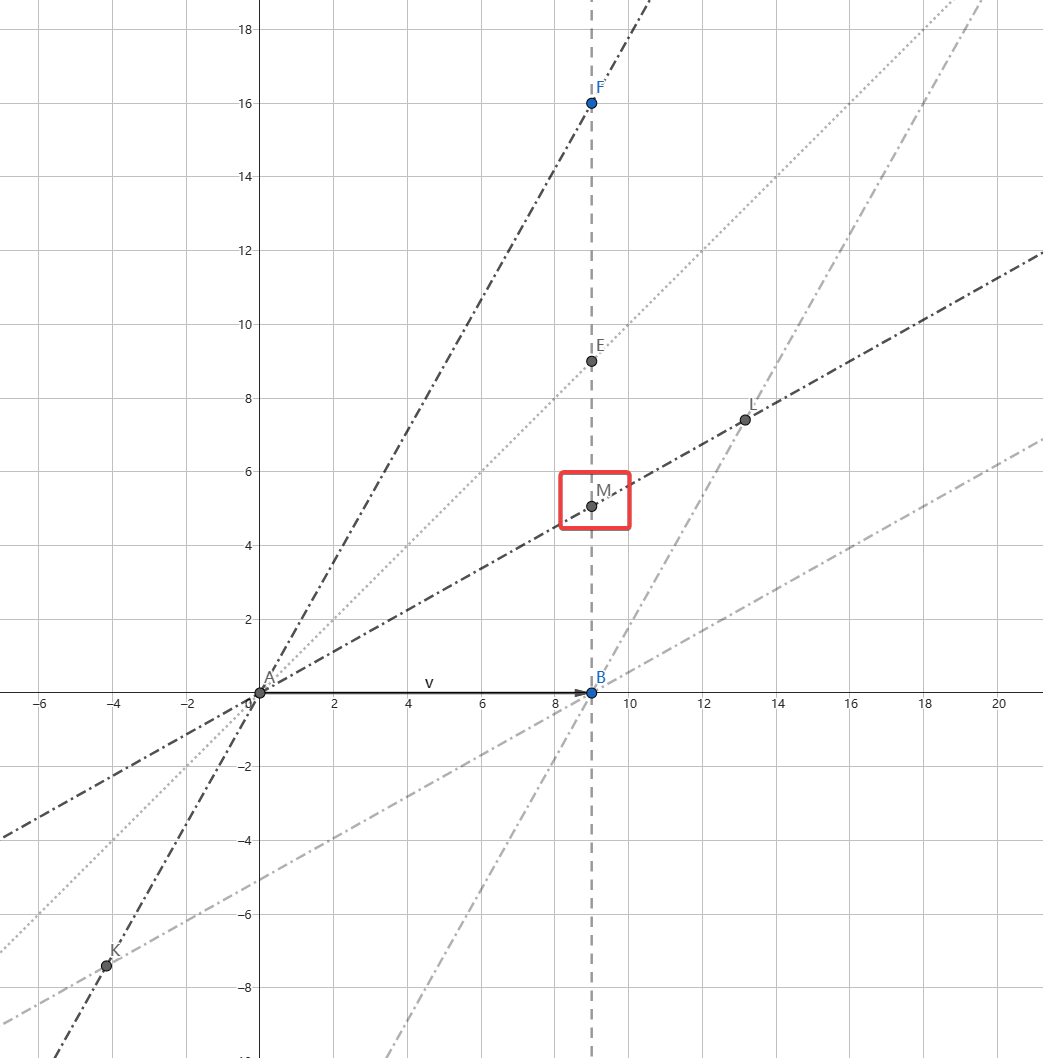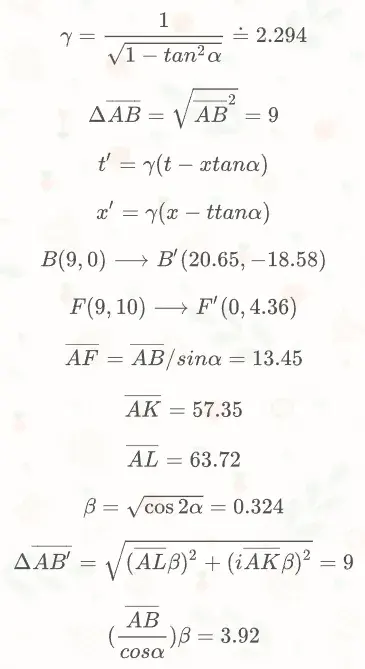# 【迁移】时空图：以天狼星单程旅行为例

Last updated on August 30, 2023 pm

## 出发前的时空图## 出发后的时空图• x’为0的直线AF为新的纵轴，t’为0的直线AL为新的横轴，两者关于AE轴对称
• AB在新坐标系的几何位置为（AL，AK），物理位置为（ALβ，AKβ）## 一些有趣的发现

1. AB的时空距离原来为 9，现在AB’的时空距离依然为 9，即 $9^2 + (0i)^2 = 20.65^2 + (-18.58i)^2$
2. AF、AL上的单位对应的几何长度均变为原来的 1/β，即 $\lvert\overrightarrow{AF}\rvert\beta = t'_{ \overrightarrow{AF'}} \quad \lvert\overrightarrow{AL}\rvert\beta = x'_{ \overrightarrow{AB'}}$
3. 对于出发的飞船上的人来说，旅行只花了 4.36 年，小于 10 年，即 $t'_{ \overrightarrow{AF'}} = 4.36 \lt 10 = t_{ \overrightarrow{AF}}$
4. 对于出发的飞船上的人来说，天狼星距离为 3.92 光年，小于9光年，B’点的天狼星是 18.58 年前的幻影，现在的天狼星是M’点的天狼星，即 $x'_{ \overrightarrow{AM'}} = (\frac{ \lvert\overrightarrow{AB}\rvert}{cos\alpha})\beta = 3.92 \lt 9 = x_{ \overrightarrow{AB}}$
5. 对于出发的飞船上的人来说，天狼星靠近的速度 v = 3.92/4.36 = 0.9 倍光速，与外界观察飞船飞行的速度一致
6. 飞船感知外界的空间长度 $x_{\overrightarrow{AB}} = 9$ 变成了空间长度 $x'_{\overrightarrow{AB'}} = \lvert\overrightarrow{AL}\rvert\beta = 20.65$，变长了γ倍，外界尺子变长了
7. 飞船感知外界的时间长度 $t_{\overrightarrow{AF}} = 10$ 变成了时间长度 $t'_{ \overrightarrow{AF'}} = \lvert\overrightarrow{AF}\rvert\beta = 4.36$，缩短为1/γ，外界时间变快了
8. 飞船认为外界“尺长钟快”，因此外界认为飞船上“尺缩钟慢”
9. 根据四维时空距离不变性，可以轻松计算飞船感知的旅程时间耗费，飞船参考系下的AF’的时空长度与外界参考系下AF的时空长度一致，即 $(i\lvert\overrightarrow{AF'}\rvert)^2 = (i\lvert\overrightarrow{AF}\rvert\beta)^2=AB^2+(iBF)^2$

【迁移】时空图：以天狼星单程旅行为例
https://hexo.limour.top/-qian-yi--shi-kong-tu--yi-tian-lang-xing-dan-cheng-l-xing-wei-li
Author
Limour
Posted on
July 5, 2022
Updated on
August 30, 2023